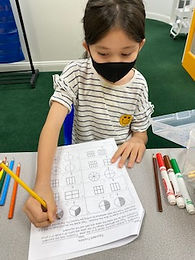## Ms. Dawn

### Target 1​

###### Lesson Type:

Continuation

Geometry

:

Modeling

Visualize spatial relationships between shapes and planar space.

###### 1:

Recognize and apply reflections (flips), translations (slides), and rotations (turns).

3rd

###### Vocabulary:

Flip, Turn, Slide, Rotate

Activities:

1. Students continued creating their picture in quadrant one. I told each student whether they will flip, slide, or turn their image in each quardrant. Students created the new image in each quadrant showing their understanding of flips, slides and turns.### Home Exploration

###### Guiding Questions:## Absent Students:

### Target 2

:

###### 1:

Compose a written fraction to describe a visual or a visual to describe a written fraction.

###### 2:

Understand and explain what a fraction is.

###### 3:

Identify the number of shaded or unshaded parts as the numerator of a fraction.

###### 4:

Identify the number of equal parts as the denominator of a fraction.

3rd

###### Vocabulary:

Fraction, Numerator, Denominator, Equivalent

Activities:

1. Students compared fractions by shading in pictures and matching it with the written form. They wrote the fraction from the visual picture and vice versa.

2. Students shaded in both circles and bars to show the fraction. Then, wrote what fractions belong to the picture.

3. Students found equivalent fractions using numbers and pictures.### Home Exploration

###### Guiding Questions:### Target 3

:

###### Vocabulary:

Activities:### Home Exploration